Visitors Online: 0 | Tuesday 10th December 2019CBSE Guess > Papers > Important Questions > Class XII > 2008 > Maths > Maths By Mrs. Geethanjali Bhatt Differential Calculus And Matrices And Determinants
Q. 1. If sin y = xcos(a + y) and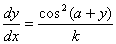find k (3 marks) Q. 2. If f(x) =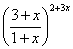find f’(o) (3marks) Q. 3. Verify the mean value theorem for f(x) =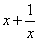in [1,3] (4 marks) Q. 4. If x = a (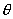+) y = (1 + cos) find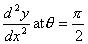(4 marks) Q. 5. If xpyq = (x + y) p+q prove that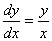Or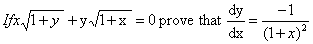(4 marks) Q. 6. Consider the function defined as follows for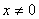. In each case ,what choice (if any) of f(0) will make the function continuous at x = 0.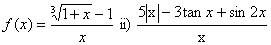(4 marks) Q. 7. If x = acos+ bsiny = asin- bcos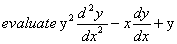(4 marks) Q. 8.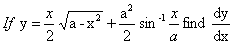Or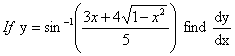(4 marks) Construct a3 x 3 matrix A = [aij]whose elements are given by aij =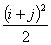( 3 marks) Solve the matrix equation for x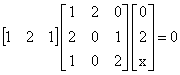(3 marks) Solve the following equation for x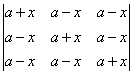(3 marks) Prove that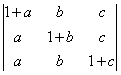= a + b + c (3 marks) Solve the following equations for using matrices  x - y + z = 4      2x + y -3z = 0      x + y + z = 2 ( 6 marks) Determinant of a skew symmetrix matrix of odd orderis always ( 1 marks)    a) zero    b) one c) negative one d) none If A is a square matrix of order 3 then A.Adj (A) is (1 marks) a) | A | I3 b) | A | I2 C) | A | I d) none